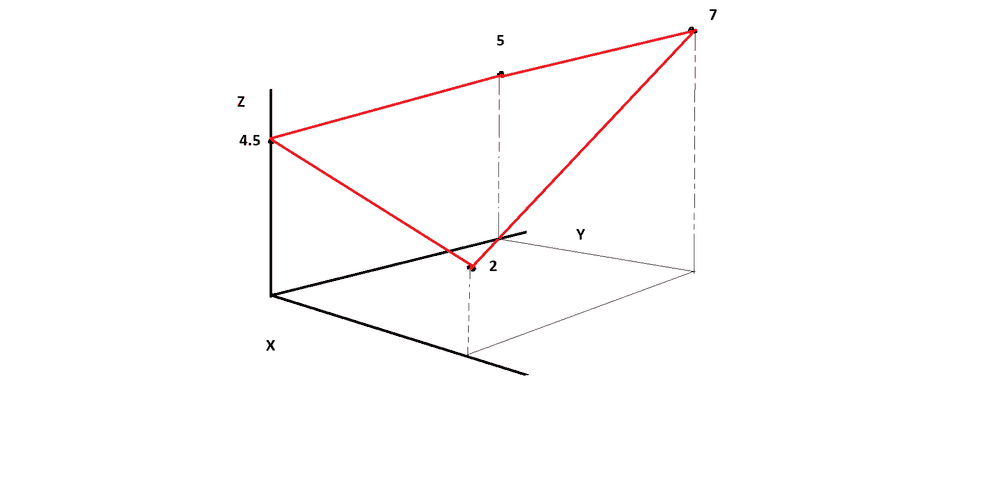# A dot in a box

• B
So I have a cube in 3d space and this cube is made out of 8 coordinate points at each corner. Now I have a temperature reading at each point of these corner points. Inside the box I have another point, I want to be able to use the information from the 8 points surrounding the the one middle point( the point in the box) to calculate the direction of change of the temperature.

So if this was in one dimension, and at p1 I had 90 degrees and p2 I had 80 degrees and I would get that the change in temperature is -10. telling me that if I go in the direction of p2 my temperature will decrease.

Someone helped me out for the case of 3d, But I don't quite understand it.

if p_1, ..., p_8 are your points and
w_1, ..., w_8 are your weights (or temperatures) and p is
the point in the middle, calculate T := Sum w_i * (p_i - p)
* (p_i - p)^T for 1 <= i <= 8, and then calculate the
eigenvectors v_1, v_2, v_3 and according eigenvalues l_1,
l_2, l_3 of T, and then pick the v_k where abs(l_k) is > 0
and the greatest or smallest of all abs(l_j) [for 1 <= j,k
<= 3]

if the point p in the middle has a temperature (w)
as well, you should to use (w_i - w) instead of w_i of course

You could also "normalize" your tensor T by multiplying with
1 / Sum w_i (or 1 / Sum (w_i - w) if you have w), but that
makes no difference for the eigenvectors

Can anybody help me?

Paul Colby
Gold Member
Well, for some value of help.

calculate T := Sum w_i * (p_i - p) * (p_i - p)^T for 1 <= i <= 8,

Is this the equation for ##T##?

## T = \sum_{i=1}^8 w_i(p_i-p)\cdot(p_i-p)##

Ah, I think T is a matrix.
## T = \sum_{i=1}^8 w_i(p_i-p)\otimes(p_i-p)##

Paul Colby
Gold Member
So to me this looks like an interpolation problem. If you choose the point (vector) ##p=p_j## the procedure should yield ##w = w_j##.

Thanks Paul. I think it is a matrix/tensor yes. 3x3. so at P_j i would get a temperature W_j? Which is a scalar. Is it possible to get a direction of the flow of the heat at point P_j? (sorry idk how to use latex)

Paul Colby
Gold Member
This is just a wild guess but what it looks like some means of building an 8'th order scalar polynomial fitting function, ##T(p,p_1,\cdots,p_8)##, which would have the properties,

##w_j=T(p_j,p_1,\cdots,p_8)##

at each node or vertex. This function could then be differentiated to yield the gradient. I just can't make it out from your writeup.Btw, I figured this problem by doing a simple 2D version, instead of a box a square. So in the above case I would figure the distances from the corners of the XY plane to the point I'm interested in on the XY plane. and then I add up these distances. after that I add distance/totalDistance*zCornerCoordinate to get where I am intersecting the plane. It is crude but it seems to work. If I want the derivative I just take a step forword in X on the XY plane and subtract that from the result. I can then extend all of this to the 3D box.

If someone has a more elegant way to do it I would like to hear.